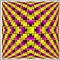# How to calculate lots in percentage118

Hi i would like to calculate lots such that if say account balance is \$1000 to open trades with \$100 in other words 10% and when the balance grows to \$2000 to open trades with \$200. This is some sort of incremental system that should maintain lot size of new trades at 10% of balance. How do i go about it its nowhere in mql4 book.91

What do mean by this? \$100 trade being how much margin is used or your maximum loss?

If it's margin then use amount/MarketInfo(Symbol(), MODE_MARGININIT). If it's maximum loss then amount/(stoploss distance * MarketInfo(Symbol(),MODE_TICKVALUE)).118

The \$100 would be trade size if say leverage is 1:100 then lot size 0.1 if my balance is \$1000 even if there is another open trade and free margin is \$900 as long as the balance is \$1000 i want it to open 10% of this. If this system is unsafe let me know.91

How you trade is entirely up to you, if your comfortable with 10% being open then trade with 10%. Personally I would go for the \$100 maximum loss rather than \$100 margin but the one you want is the first one: amount/MarketInfo(Symbol(), MODE_MARGININIT). And you would want to use AccountBalance()

```double lots = MarketInfo(Symbol(), MODE_MINLOT),money=AccountBalance()*(10/100);
lots =(money/MarketInfo(Symbol(), MODE_MARGININIT));
Print("Not enough margin to open even the smallest size lot");
}```

Try that.118

Ill try it thanks alot118

Journal gives error "zero divide"1863

jameslarry:
Hi i would like to calculate lots such that if say account balance is \$1000 to open trades with \$100 in other words 10% and when the balance grows to \$2000 to open trades with \$200. This is some sort of incremental system that should maintain lot size of new trades at 10% of balance. How do i go about it its nowhere in mql4 book.

Compiled, not tested not checked, take your own risk, use wisely.

```double Last_Account_Balance, Money_Use_To_Trade, Value_Per_Point, SL,
Loss_By_SL, Lot;

int start()
{
if (AccountBalance() >= 1000 + Last_Account_Balance)
{
Last_Account_Balance = AccountBalance();  // starting balance to trade
}

if (AccountBalance() < Last_Account_Balance)
{
//--- account protection
Print ("Your balance is lower than the last one.");
return(0);
}
else
{
if (Last_Account_Balance > 0)
{
//--- calculating loss
Value_Per_Point = MarketInfo(Symbol(), MODE_TICKVALUE) / (MarketInfo (Symbol(), MODE_TICKSIZE)/ Point);
if (SL  <= 0) SL = 100;
Loss_By_SL = SL * Value_Per_Point;
Lot = NormalizeDouble(Money_Use_To_Trade / (Loss_By_SL+ MarketInfo (Symbol(), MODE_MARGINREQUIRED)), 2);
if (Lot < MarketInfo (Symbol(), MODE_MINLOT))
{
Print ("You can\'t afford to open the smallest lot.");
return(0);
}
else
{
Lot -= MarketInfo (Symbol(), MODE_MINLOT);
int size_lot;
if (Lot >= MarketInfo (Symbol(), MODE_LOTSTEP))
size_lot = Lot / MarketInfo (Symbol(), MODE_LOTSTEP);
Lot = MarketInfo (Symbol(), MODE_MINLOT)+(size_lot* MarketInfo (Symbol(), MODE_LOTSTEP));
}

if (Lot > MarketInfo(Symbol(), MODE_MAXLOT))
{
Lot = MarketInfo(Symbol(), MODE_MAXLOT);
}
}
}

return(0);
}```118

I use EURUSD and this is the pair im interested in20233

jameslarry:
Hi i would like to calculate lots such that if say account balance is \$1000 to open trades with \$100 in other words 10% and when the balance grows to \$2000 to open trades with \$200. This is some sort of incremental system that should maintain lot size of new trades at 10% of balance. How do i go about it its nowhere in mql4 book.
Contract Size - MQL4 forum1863

onewithzachy:

Compiled, not tested not checked, take your own risk, use wisely.

The reason I said that, was because I did not calculate any Swaps, AccountStopoutLevel (), AccountStopoutMode(), because that will involve more calculation.

Here, this is an example on how to calculate the price 1 lot of EURUSD. For example the current price EURUSD is 1.23456, leverage is 500, so the price of 1 standard lot of EURUSD is (1.23456 * 100.000)/500 which is the same with MarketInfo(Symbol(), MODE_MARGINREQUIRED).

Just open 1 lot of EURUSD and see it your self.

Files:118

onewithzachy:

The reason I said that, was because I did not calculate any Swaps, AccountStopoutLevel (), AccountStopoutMode(), because that will involve more calculation.

Here, this is an example on how to calculate the price 1 lot of EURUSD. For example the current price EURUSD is 1.23456, leverage is 500, so the price of 1 standard lot of EURUSD is (1.23456 * 100.000)/500 which is the same with MarketInfo(Symbol(), MODE_MARGINREQUIRED).

Just open 1 lot of EURUSD and see it your self.

Ok ill check it out and reply